# Prime Numbers

## Mathematician James Maynard on the Riemann Hypothesis, Euclid’s proof, and the problems of Paul Erdős

### faq | June 28, 2016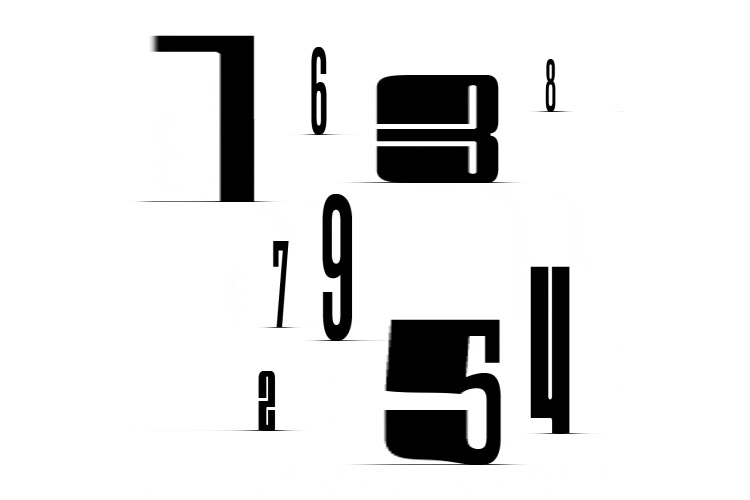Prime numbers are whole numbers (1,2,3, and so on) bigger than 1, which have the property that they can’t be written as two smaller numbers multiplied together. So 6 isn’t prime since it can be written as 2×3, but 5 is prime since the only way of writing it as two whole numbers multiplied together is 1×5 or 5×1. Another way of thinking about this is if you have some coins, but you can’t arrange all of them into a rectangle other than by putting them in a straight line, then the number of coins is a prime number.

### An Infinite Number Of Primes

It might sound strange why anyone would care about such numbers, but it turns out they’re fundamental to mathematics. This is because every number can be written in a unique way, as prime numbers are multiplied together. This means that prime numbers are the `atoms of multiplication’ – the smallest things which everything else can be built from.

Because primes are the building blocks of whole numbers by multiplication, many problems about whole numbers can be reduced to a problem about prime numbers. In much the same way, some problems in Chemistry can be solved by understanding the atomic composition of the chemicals involved. Thus, if there were a finite number of primes, one could possibly then check each one in turn on a computer. However, it turns out that there are infinitely many prime numbers and that prime numbers are still surprisingly poorly understood by mathematicians.Mathematician Gilbert Strang on the difference between cosine and wavelet functions, audio compression, and the JPEG format
The fact that there are infinitely many prime numbers is a famous result of the Greek mathematician Euclid, who gave beautiful proof of the result. If you have a list of prime numbers – let’s call them p1,…,pn – then you can consider the number p1×…×pn + 1, which is one more than all these primes multiplied together. This number cannot be a multiple of any of the primes p1,…,pn from your list, but it is clearly bigger than 1. So all its prime factors must be new primes that aren’t on your list. By adding these new primes to your list and repeating them, you can always find at least one new prime, so there must be infinitely many prime numbers.

### History of Research

Nobody quite knows who were the first societies to consider prime numbers – they go back so far that we don’t have accurate records of that time. There is speculation that some of the very earliest human civilizations had some concept of primes, but the first concrete evidence appears to be some Papyrus writings of the ancient Egyptians from over 3500 years ago.

The ancient Greeks were probably the first to study the primes as an object of academic interest in their own right, and they appreciated the importance of prime numbers to pure abstract mathematics. Euclid’s proof above is one of my favorite proofs and is still taught today in schools despite being over 2000 years old.

After the Greeks, prime numbers only really started getting serious attention again in the 17th century. Since then, many of the most famous mathematicians have made important contributions to our understanding of primes. Pierre de Fermat made many discoveries and is famous these days for `Fermat’s last theorem’, a 350-year-old problem connected to primes that were solved by Andrew Wiles just 20 years ago. Leonhard Euler proved many results in the 18th century, and in the 19th century, there were huge advances due to Carl Friedrich Gauss, Pafnuty Chebyshev, and Bernhard Riemann, particularly on the distribution of primes. This culminated with the still unsolved `Riemann Hypothesis’, often referred to as the most important unsolved problem in all of mathematics. The `Riemann Hypothesis’ gives a very precise prediction of how often primes occur, as well as explains in part why they are so difficult for mathematicians to study.

### Practical Applications

Prime numbers have a huge number of applications, both within mathematics and in the wider world. In fact, these days, primes are used by all of us on an almost daily basis, even if we’re not aware of it at the time.

For mathematicians, primes are important because they are the atoms of multiplication, so many abstract problems involving multiplication can be solved if we know enough about prime numbers. It is common for mathematicians to break a problem down into many smaller ones, and the primes would be a great way of doing this – if only we understood them a bit better.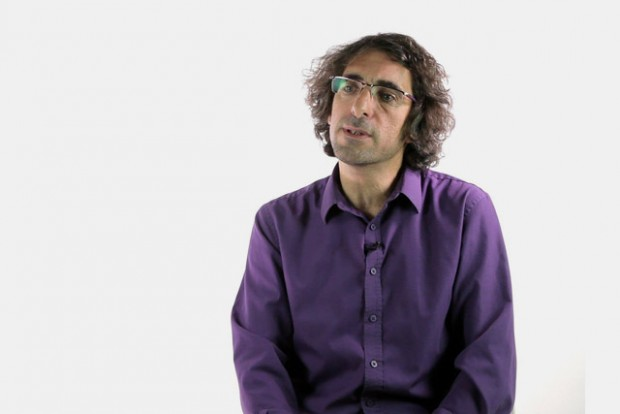Mathematician Itzhak Gilboa on known and unknown probabilies, various descriptive models, and ambiguity
In the wider world, the main uses of prime numbers are related to computers. Computers store all data as a string of ‘0’s and ‘1’s, which can be interpreted as a whole number. Many computer programs need to multiply the numbers associated with the data together, which means that primes are lying just below the surface. Whenever you buy something securely online, you use the fact that there are ways to multiply numbers together, which are very hard for a potential hacker to reverse, but easy for you to reverse. This relies on the fact that primes don’t have some special properties – if they did, someone might be able to hack all your credit card details.

### Finding New Primes

One way to find prime numbers is to ask a computer to look for them. A simple way to check if a number is prime is to repeatedly see if it is a multiple of 2, or a multiple of 3, a multiple of 4, and so on. If it is not a multiple of any smaller number, then it is prime. It turns out this is a very wasteful way of checking if a number is prime, but there are very efficient ways of testing to see if a number is prime. The fact that these algorithms are efficient for every number is a relatively recent theoretical breakthrough from 2002, so we’re still making progress even after 3000 years.

Fortunately, primes are quite plentiful, so if you take a large number and then keep on adding one, it won’t be too long before you encounter a prime number. Indeed, many computer programs rely on the fact that it isn’t too hard to find prime numbers. This means that if you choose a random number with 100 digits, say, your computer can find the next prime bigger than it in a few seconds. Since there are more 100-digit prime numbers than there are atoms in the universe, it is also very likely that no one will have explicitly known that this number was prime, so you’ll have discovered a new prime.

Mathematicians typically aren’t interested in finding individual primes on a computer but are very interested in finding primes with special properties. Two famous problems are whether there are infinitely many primes that are one more than a square (this has consequences in a different area of mathematics known as group theory, for example) or if there are infinitely many pairs of primes that differ by 2.

### Mysteries of Prime Numbers

Despite being studied for over 3000 years and having a pretty simple description, we still know surprisingly little about prime numbers. For example, we know that the only pair of prime numbers which differ by 1 is the primes 2 and 3, but we don’t know if there are finitely or infinitely many pairs that differ by 2 We guess infinitely many – but we can’t prove it. This is a problem that can be explained to a school child but has thwarted the greatest mathematical minds for over 100 years.

Many of the most interesting questions about primes from both a practical and a theoretical point of view are questions that ask how many primes are there with a certain property. The simplest such question is how many primes there are of a certain size – which would be theoretically answered by a solution to the Riemann Hypothesis. An added incentive for proving the Riemann Hypothesis is the \$1 million prize offered by the Clay Foundation, as well as eternal mathematical fame.Biomedical Engineer Thomas Heldt on mathematical dimension of physiology, organ systems multiscale modelling, and its practical application
We now have good ways of guessing what the right answer should be too many of these questions. So far our guesses have passed every numerical experiment, and we have good theoretical reasons for believing them, so we’re quite confident. However, it is of vital importance for pure mathematics and the working of computer algorithms that these guesses are indeed correct, and mathematicians are only ever satisfied by rigorous proof.

For applications to the real world, the biggest challenge is how easy it is to find all the prime factors of a number. Given the number 15, it won’t take you long to realize that 15=5×3. But given a 1000-digit number, it might take the fastest supercomputer in the world over a billion years to find its prime factors. A huge amount of internet security relies on the fact that this is very difficult, so it is very important for the security of communication that we don’t think someone can just come up with a very quick way to do this.

### Modern Research

Right now is a great time for prime number discoveries. Even though the subject is so old and involves many of the most famous people in the history of mathematics, the subject is really alive and buzzing.

It isn’t known whether there are infinitely many pairs of prime numbers like 3 and 5, which differ by 2, and this is a famous unsolved problem. However, huge progress on this problem was made just a couple of years ago by Yitang Zhang. At the beginning of 2013, we didn’t know if there were infinitely many pairs of prime numbers that were within 1 billion billion of each other or for any fixed number in place of 1 billion billion, no matter how large. We now know, thanks to theoretical advances building on Zhang’s work, that there are infinitely many pairs of primes that differ by no more than 246. Two hundred forty-six might be quite a bit bigger than 2, but it is a lot smaller than infinity.

Instead of looking for primes close together, you could look for primes that are far from other primes on the number line. Noticeable theoretical improvements on this problem we made for the first time in over 75 years at the beginning of 2014 in joint work with myself and four other mathematicians, and in doing so we solved a problem of Paul Erdős. Two other exciting solutions to problems of Erdős related to prime numbers were solved over the past 3 years by Bob Hough and Terence Tao, whose work built on another breakthrough of Kaisa Matomaki and Maksym Radziwill from 2014. Harald Helfgott, with help from David Platt, culminated a century of results to finally fully prove Goldbach’s weak conjecture. We’re used to having to wait a decade for a big result in prime numbers, but we’ve had half a dozen in the past three years.

### Prime Numbers in the Future

It is impossible to tell how prime numbers can be useful in the future. Pure mathematics (such as the study of prime numbers) had repeatedly turned out to have uses that were completely impossible to envisage when the theory was first developed. Time and time again, things that seemed to be an odd theoretical interest with no real-world uses have turned out to be amazingly useful for science and technology. G. H. Hardy, a famous mathematician of the early 20th century, claimed that prime numbers had no real-world application. Forty years later, their potential use in computer communication was discovered, and now they are vitally important to our everyday internet activities.

Since prime numbers are so central and fundamental to problems involving whole numbers, and whole numbers appear all the time in real-world situations, prime numbers will definitely find applications all over the place in future society. This will be particularly true as we move to a more connected world with ever bigger roles being played by technology and computers.

There is some belief that aspects of prime numbers and number theory extend well beyond just the confines of science and computers. In music, prime numbers can explain why some complex rhythms take a long time to repeat, and this is used in some modern classical music for a particular sonic effect. The Fibonacci sequence appears in many places in nature, and it has been suggested that cicadas have evolved to hibernate for a prime number of years for evolutionary advantage. It has been suggested that the best way to attempt to communicate with alien life forms would be to broadcast prime numbers by radio waves, as primes are independent of any concept of language but suitably complicated that they couldn’t be mistaken for the output of some purely physical process of nature.

Edited by Alina ShubinaPhD, Research Fellow, Mathematical Institute, University of Oxford
Did you like it? Share it with your friends!
Published items
0779
To be published soon
+92

#### Most viewed

• 1Peer Fischer
• 2Diana Omigie
• 3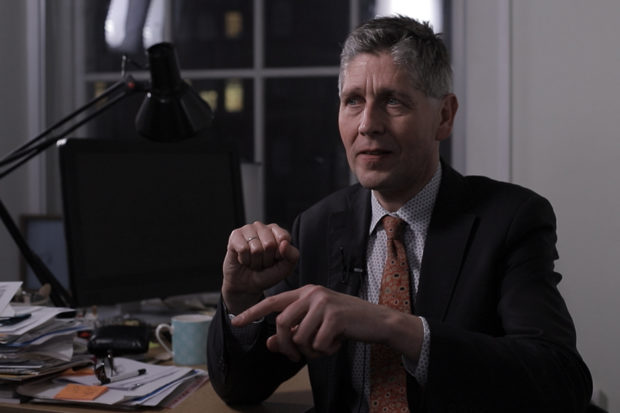Patrick Haggard
• 4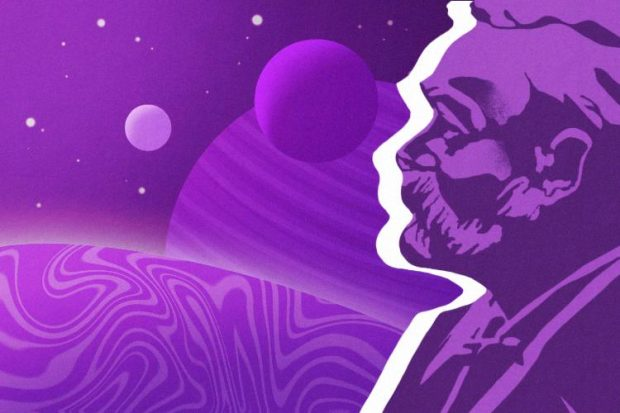Ugo Moschella
• 5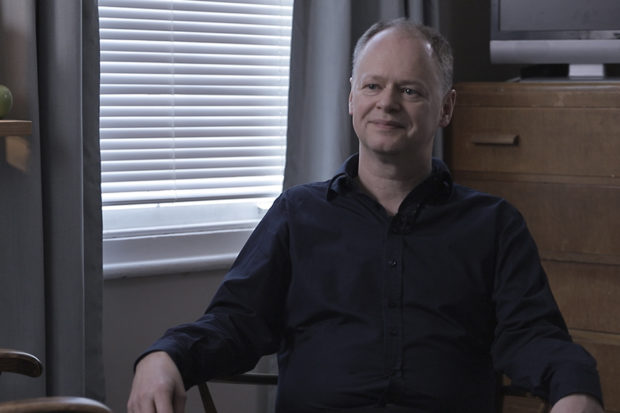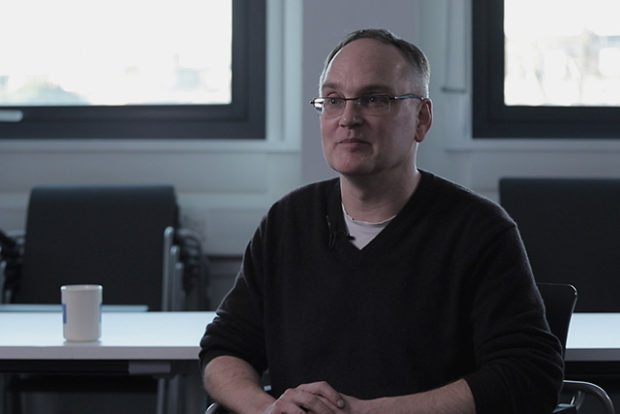•